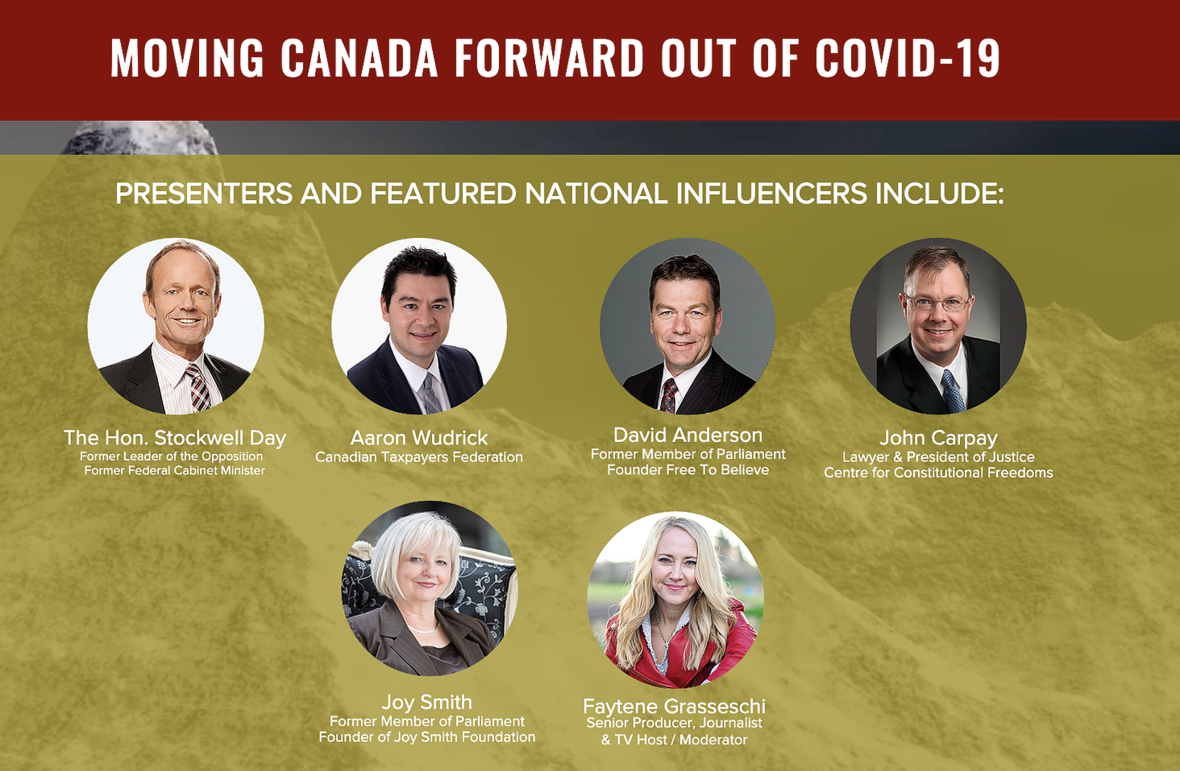Like   Tweet   Pin   +1   in
 /* styles */ May 25, 2020,
 /* styles */ Dear Fellow Canadians, As our governmental leaders grapple with how to protect Canadians from a potential 2nd wave of COVID-19, front and centre in the plan has been the push for a vaccination. At the same time some jurisdictions are already overtly, or subtly, edging towards making vaccinations mandatory in order for Canadians to access some public services & benefits. See this article from the Saskatchewan Herald: CALLS COME FOR CANADA TO MAKE COVID-19 VACCINE MANDATORY FOR ALL CITIZENS WHILE PROTECTING CIVIL RIGHTS The question remains as to which jurisdictions in Canada may edge this direction and which vaccinations may be included in the "mandatory" category. One thing is clear: the conversation is on.Faytene on her FB live about this issue.

# BILL 11 AND NEW BRUNSWICK

To add urgency to the conversation, New Brunswick is expected to vote soon on a mandatory vaccination bill for children attending public school (Bill 11).

Tomorrow, Tuesday May 26th, the legislature will decide if this bill goes to the next phase or not (committee).

A rally is planned tomorrow at the NB Legislative Assembly in Fredericton at 12:30pm.

The rally may be postponed if the sitting is postponed. Plan to go but stay tuned at at the event FB page here.

# Help Us Stand For Canada

 table div table+table+table+table+table+table div table{width:100%;padding:0}table div table+table+table+table+table+table div table img{width:96.23%;padding:0;float:none}table div table+table+table+table+table+table div table td{width:100%;padding:0 1.88% 18px}/* styles */table div table+table+table+table+table+table+table div table,table.module-6{width:48.68%;float:left;padding:0}table div table+table+table+table+table+table+table div table a{border:0 none;text-decoration:none}table div table+table+table+table+table+table+table div table img{width:100%!important;border:0 none;text-decoration:none}table div table+table+table+table+table+table+table div table td{width:100%;padding:0 20px 20px 0}/* styles */ Your generosity helps us keep in the fight for Canada. Thank you. Donations can also be send by e-mail to admin@4mycanada.ca
 table div table+table+table+table+table+table+table+table div table{width:100%;padding:0}table div table+table+table+table+table+table+table+table div table img{width:96.23%;padding:0;float:none}table div table+table+table+table+table+table+table+table div table td{width:100%;padding:0 1.88% 18px}/* styles */## Featured Media Clips

 table div table+table+table+table+table+table+table+table+table+table div table{width:100%;padding:0}table div table+table+table+table+table+table+table+table+table+table div table img{width:96.23%;padding:0;float:none}table div table+table+table+table+table+table+table+table+table+table div table td{width:100%;padding:0 1.88% 18px}/* styles */table div table+table+table+table+table+table+table+table+table+table+table div table td,table.module-10{width:100%;padding:0}table div table+table+table+table+table+table+table+table+table+table+table div table{width:100%;float:none;margin-left:auto;margin-right:auto;padding:0}table div table+table+table+table+table+table+table+table+table+table+table div table a{border:0 none;text-decoration:none}table div table+table+table+table+table+table+table+table+table+table+table div table img{width:100%!important;border:0 none;text-decoration:none}/* styles */
 table div table+table+table+table+table+table+table+table+table+table+table+table div table{width:100%;padding:0}table div table+table+table+table+table+table+table+table+table+table+table+table div table img{width:96.23%;padding:0;float:none}table div table+table+table+table+table+table+table+table+table+table+table+table div table td{width:100%;padding:0 1.88% 18px}/* styles */table div table+table+table+table+table+table+table+table+table+table+table+table+table div table,table.module-12{width:32.08%;float:right;padding:0}table div table+table+table+table+table+table+table+table+table+table+table+table+table div table a{border:0 none;text-decoration:none}table div table+table+table+table+table+table+table+table+table+table+table+table+table div table img{width:100%!important;border:0 none;text-decoration:none}table div table+table+table+table+table+table+table+table+table+table+table+table+table div table td{width:100%;padding:0 0 20px 20px}/* styles */ Check out Faytene.tv for episodes on key issues facing our nation. Click here to go there now and view recent episodes.
 table div table+table+table+table+table+table+table+table+table+table+table+table+table+table div table{width:100%;padding:0}table div table+table+table+table+table+table+table+table+table+table+table+table+table+table div table img{width:96.23%;padding:0;float:none}table div table+table+table+table+table+table+table+table+table+table+table+table+table+table div table td{width:100%;padding:0 1.88% 18px}/* styles */## ANNOUNCEMENTS //

 table div table+table+table+table+table+table+table+table+table+table+table+table+table+table+table+table div table{width:100%;padding:0}table div table+table+table+table+table+table+table+table+table+table+table+table+table+table+table+table div table img{width:96.23%;padding:0;float:none}table div table+table+table+table+table+table+table+table+table+table+table+table+table+table+table+table div table td{width:100%;padding:0 1.88% 18px}/* styles */## REGISTRATION FREE AND NOW OPEN!

 table div table+table+table+table+table+table+table+table+table+table+table+table+table+table+table+table+table+table div table td,table.module-17{width:100%;padding:0}table div table+table+table+table+table+table+table+table+table+table+table+table+table+table+table+table+table+table div table{width:100%;float:none;margin-left:auto;margin-right:auto;padding:0}table div table+table+table+table+table+table+table+table+table+table+table+table+table+table+table+table+table+table div table a{border:0 none;text-decoration:none}table div table+table+table+table+table+table+table+table+table+table+table+table+table+table+table+table+table+table div table img{width:100%!important;border:0 none;text-decoration:none}/* styles */Canada Summit Online 2020 is June 13 & 27th. Let's create the change our nation needs.

Speakers for this year's Canada Summit online will include:

▪ The Hon. Stockwell Day, Former Leader of the Opposition
▪ Joy Smith, Former MP and Founder of the Joy Smith Foundation
▪ John Carpay, Justice Centre for Constitutional Freedoms
▪ David Anderson, Former MP and Founder of Free to Believe
▪ Aaron Wudrick, Federal Director / Canadian Taxpayers Federation
 ▪ The Hon. Stockwell Day, Former Leader of the Opposition
 ▪ Joy Smith, Former MP and Founder of the Joy Smith Foundation
 ▪ John Carpay, Justice Centre for Constitutional Freedoms
 ▪ David Anderson, Former MP and Founder of Free to Believe
 ▪ Aaron Wudrick, Federal Director / Canadian Taxpayers Federation

These speakers (and others) will be talking about how to rebuild Canada coming out of COVID-19 with an emphasis on:

▪ Our Economy
▪ Freedom Rights
▪ Domestic Strength
▪ Healthcare Preparedness
 ▪ Our Economy
 ▪ Freedom Rights
 ▪ Domestic Strength
 ▪ Healthcare Preparedness

We hope you can join. Registration is FREE for this year.

Register now.

Space for the innovation sessions are limited.

 table div table+table+table+table+table+table+table+table+table+table+table+table+table+table+table+table+table+table+table+table+table+table div table td,table.module-21{width:100%;padding:0}table div table+table+table+table+table+table+table+table+table+table+table+table+table+table+table+table+table+table+table+table+table+table div table{width:100%;float:none;margin-left:auto;margin-right:auto;padding:0}table div table+table+table+table+table+table+table+table+table+table+table+table+table+table+table+table+table+table+table+table+table+table div table a{border:0 none;text-decoration:none}table div table+table+table+table+table+table+table+table+table+table+table+table+table+table+table+table+table+table+table+table+table+table div table img{width:100%!important;border:0 none;text-decoration:none}/* styles */
 table div table+table+table+table+table+table+table+table+table+table+table+table+table+table+table+table+table+table+table+table+table+table+table div table{width:100%;padding:0}table div table+table+table+table+table+table+table+table+table+table+table+table+table+table+table+table+table+table+table+table+table+table+table div table img{width:96.23%;padding:0;float:none}table div table+table+table+table+table+table+table+table+table+table+table+table+table+table+table+table+table+table+table+table+table+table+table div table td{width:100%;padding:0 1.88% 18px}/* styles */